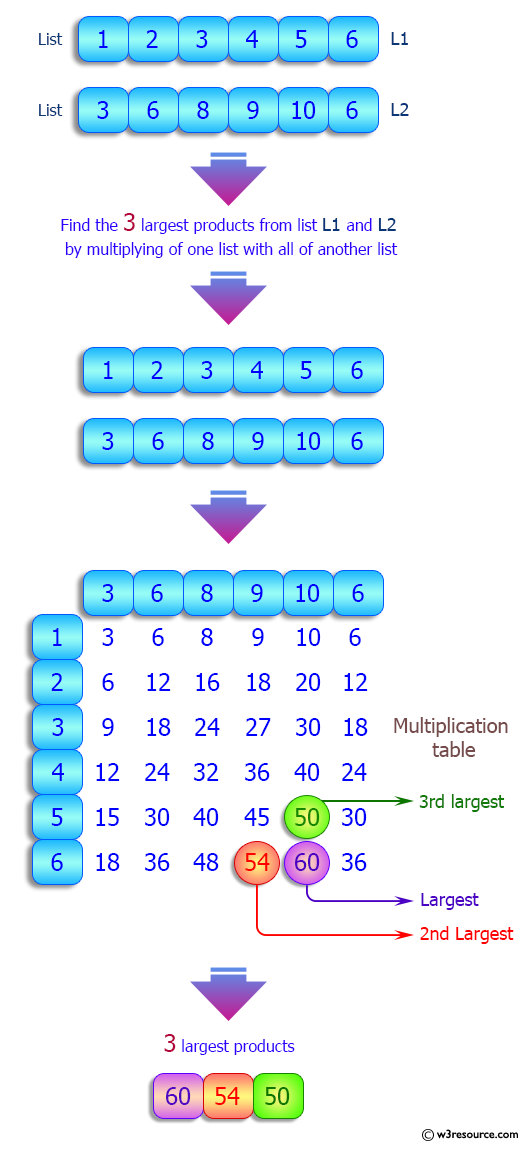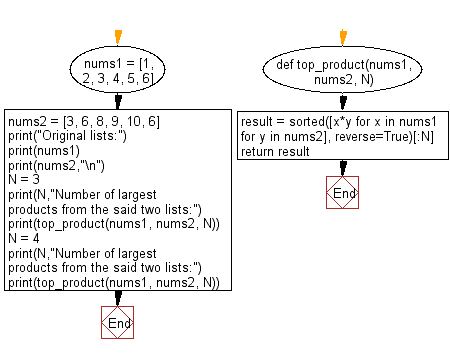﻿ Python: Find the specified number of largest products from two given list, multiplying an element from each list - w3resource# Python: Find the specified number of largest products from two given list, multiplying an element from each list

## Python List: Exercise - 190 with Solution

Write a Python program to find the specified number of largest products from two given list, multiplying an element from each list.

Sample Solution:

Python Code:

``````def top_product(nums1, nums2, N):
result = sorted([x*y for x in nums1 for y in nums2], reverse=True)[:N]
return result
nums1 = [1, 2, 3, 4, 5, 6]
nums2 = [3, 6, 8, 9, 10, 6]
print("Original lists:")
print(nums1)
print(nums2,"\n")
N = 3
print(N,"Number of largest products from the said two lists:")
print(top_product(nums1, nums2, N))
N = 4
print(N,"Number of largest products from the said two lists:")
print(top_product(nums1, nums2, N))
```
```

Sample Output:

```Original lists:
[1, 2, 3, 4, 5, 6]
[3, 6, 8, 9, 10, 6]

3 Number of largest products from the said two lists:
[60, 54, 50]
4 Number of largest products from the said two lists:
[60, 54, 50, 48]
```

Pictorial Presentation:Flowchart:## Visualize Python code execution:

The following tool visualize what the computer is doing step-by-step as it executes the said program:

Python Code Editor:

Have another way to solve this solution? Contribute your code (and comments) through Disqus.

What is the difficulty level of this exercise?

Test your Python skills with w3resource's quiz

﻿

## Python: Tips of the Day

Floor Division:

When we speak of division we normally mean (/) float division operator, this will give a precise result in float format with decimals.

For a rounded integer result there is (//) floor division operator in Python. Floor division will only give integer results that are round numbers.

```print(1000 // 300)
print(1000 / 300)```

Output:

```3
3.3333333333333335```